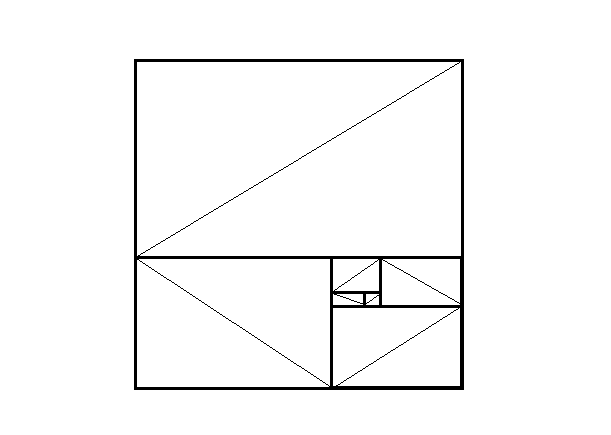# Inspired by Otto...Funny back to the futureThe Fibonacci sequence is defined with the recurrence relation $f_{n} = f_{n-1} + f_{n-2}$ for $n>2$ with initial terms $f_1 = 1$, $f_2 = 1$.

$(1 \cdot 1) + (1 \cdot 2) + (2 \cdot 3) + (3 \cdot 5) + \ldots + (f_{2012} \cdot f_{2013}) + (f_ {2013} \cdot f_{2014})$

If the value of the expression above equals $f_n ^2$, find the value of $n$.

×